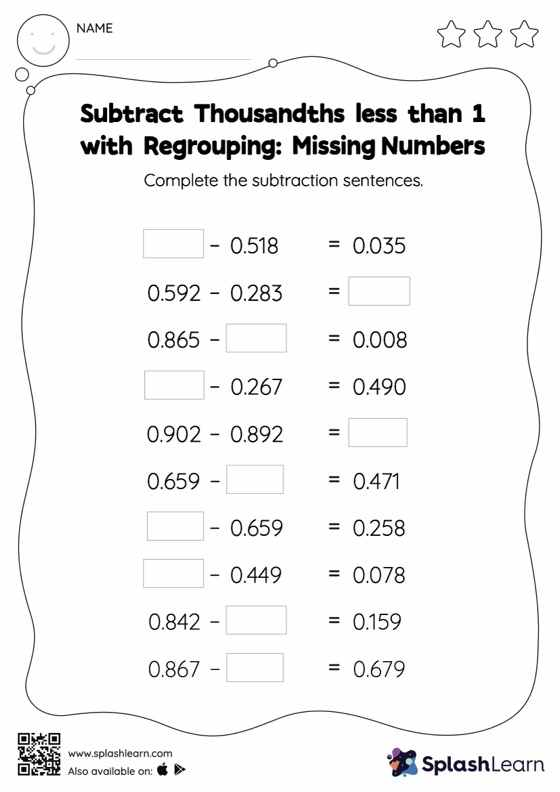# Subtract Thousandths less than 1 with Regrouping: Missing Numbers Worksheet

Home > Subtract Thousandths less than 1 with Regrouping: Missing NumbersThis subtract thousandths less than 1 with regrouping worksheet consists of a set of questions on subtraction, which help students develop fluency. While subtracting decimals, students use the relationship between addition and subtraction to find the missing number. They also need to regroup numbers in subtract thousandths less than 1 with regrouping worksheet utilizing the relationship between ones, tenth, hundredth, thousandth, etc., To get to the result.